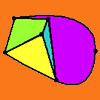# Resource Title search

#### Text Search of NRICH resource titles

##### Or find resource begining with:### Equations and Formulae

##### Age 11 to 16 Challenge Level:

The equations and formulae collection of STEM resources### Equations and Formulae### Equations and Formulae - Short Problems

##### Age 11 to 16

A collection of short problems on equations and formulae.### Equations and Formulae - Stage 3

Working on these Stage 3 problems will help you develop a better understanding of equations and formulae.### Equations and Formulae - Stage 4

Working on these problems will help you develop a better understanding of equations and formulae.### Equations and Formulae: Age 11-14

Working on these problems will help your students develop a better understanding of equations and formulae.### Equations and Formulae: Age 14-16

Working on these problems will help your students develop a better understanding of equations and formulae.### Euler's Buckling Formula

##### Age 16 to 18 Challenge Level:

Derive Euler's buckling formula from first principles.### Euler's Formula

##### Age 16 to 18

Some simple ideas about graph theory with a discussion of a proof of Euler's formula relating the numbers of vertces, edges and faces of a graph.### Euler's Formula and Topology

##### Age 16 to 18

Here is a proof of Euler's formula in the plane and on a sphere together with projects to explore cases of the formula for a polygon with holes, for the torus and other solids with holes and the. . . .### Proving Half-angle Formulae

##### Age 16 to 18 Challenge Level:

Can you find a geometric proof of these half-angle trig identities?